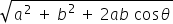science
Easy

Question

# Two vectors started from the origin and moved to the east and north direction 5 m, 7 m, respectively, and the angle between the vectors is 90 degrees, then find the resultant of the vectors.

## 21.31.58.6Hint:

## The correct answer is: 8.6

science

### Two vectors started from the origin and moved to the east and north direction 10 m, 15 m, respectively, and the angle between the vectors is 30 degrees, then find the resultant of the vectors.

If two vectors A and B with magnitude a and b respectively are given and the angle between them is θ Then the magnitude of the Resultant of A and B is R =### Two vectors started from the origin and moved to the east and north direction 10 m, 15 m, respectively, and the angle between the vectors is 30 degrees, then find the resultant of the vectors.

If two vectors A and B with magnitude a and b respectively are given and the angle between them is θ Then the magnitude of the Resultant of A and B is R =science

science

### If a vector has magnitude 1, then the vector is called asscience

science

science

### The displacement in a closed curve isscience

science

science

### The angle in the triangle law is represented byscience

science

science

### If the vectors are of the same magnitude and same direction, they are said to be

Two vectors are said to be equal if they have same magnitude and direction, regardless of the positions of their positions of their initial points.

### If the vectors are of the same magnitude and same direction, they are said to be

Two vectors are said to be equal if they have same magnitude and direction, regardless of the positions of their positions of their initial points.science

### The angle between the vectors is _______ if they are orthogonal

Two vectors are orthogonal when they are perpendicular to each other. i.e. 90 degrees and the dot product of the two vectors is 0.

### The angle between the vectors is _______ if they are orthogonal

Two vectors are orthogonal when they are perpendicular to each other. i.e. 90 degrees and the dot product of the two vectors is 0.

science

science

### Angle in the graphical representation represents

If the length of the arrow gives the magnitude of the vector, then the angle would give the direction of the vector quantity.

### Angle in the graphical representation represents

If the length of the arrow gives the magnitude of the vector, then the angle would give the direction of the vector quantity.#### With Turito Foundation.#### Get an Expert Advice From Turito.